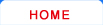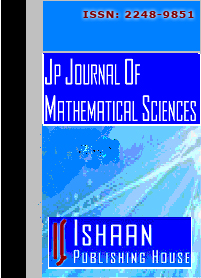Journal HomeEditorial BoardGuidelines for AuthorsSubscriptionContentOnline SubmissionJP Journal of Mathematical Sciences Editorial Board
 Ahmad, Bashir Department of Mathematics Faculty of Science King Abdulaziz University P. O. Box 80203 , Jeddah 21589 Saudi Arabia [Ordinary differential equations, Boundary value problems, Wave motion] Ahmed, Mohamed M. Eldessoky Department of Mathematics Faculty of Science Mansoura University Mansoura, 35516 Egypt Ansari, A. R. Department of Mathematics & Natural Sciences Gulf University for Science & Technology P. O. Box 7207, Hawally 32093 Kuwait [Numerical methods for problems with boundary layers, Computational fluid dynamics, Perturbation and other perturbation type methods for solving differential equations specifically applied to problems in fluid mechanics, Biological fluid mechanics] Azari, Hossein Department of Mathematical Sciences Shahid Beheshti University Tehran Iran [♦ Partial Differential Equations: Inverse problems, Non–local problems, Partial integro–differential equations, Coupled PDE’s. ♦ Numerical Analysis: Finite difference, Finite element, Finite volume element, Collocation method analysis, Computations. ♦ Industrial Mathematics Reservoir Simulation, Porous media fluid flows, Image processing, Mathematical finance, Option pricing] Baleanu, Dumitru Department of Mathematics and Computer Science Çankaya University 06530 Ankara Turkey [ODE, Fractional calculus, Fractional dynamics] Bayoumi, Fatma Department of Mathematics Faculty of Science Benha University Benha 13518 Egypt [Fuzzy Topology, It could be topics related to fuzzy theory or to general topology] Chang, Qian-Shun Institute of Applied Mathematics Academy of Mathematics and Systems Science Beijing, 100190 P. R. China [Numerical methods for differential equations, Image processing] Fazio, G. Di Dipartimento di Matematica e Informatica Università di Catania Viale A. Doria 6, 95125 Catania Italy [PDE especially elliptic, Harmonic Analysis related to PDE] Gavriluţ, Alina Department of Mathematics Faculty of Mathematics "Al. I. Cuza" University from Iaşi Carol I, Bd. 11, Iaşi 700506 Romania [Mathematics - Analysis - (fuzzy) measure theory] Jerry, M. Département d’économie Faculté Polydisciplinaire de Béni Mellal Université sulran Moulay Slimane Maroc [Optimal control, Application of viability theory and application of dynamical systems] Jumarie, Guy Department of Mathematics University of Québec at Montréal P. O. Box 8888, Downtown Station Montréal, Qc H3C 3P8 Canada [Fractal and fractional calculus together with their applications for instance Stochastic processes, Hamiltonian mechanics, Relativity, Differential geometry] Korotov, Sergey Institute of Mathematics Helsinki University of Technology P. O. Box 1100, FI-02015 TKK Helsinki Finland [Numerical mathematics] Kórus, P. Bolyai Institute University of Szeged H-6720 Szeged, Aradi vértanúk tere 1 Hungary [Fourier analysis] Lagodowski, A. Department of Mathematics Lublin University of Technology Nadbystrzycka 38d, 20-618 Lublin Poland [Probability is main area of my interest with special with emphasis on limit theorems] Li, Ji-Bin Department of Mathematics Zhejiang Normal University Zhejiang, 321004 P. R. China [Dynamical systems, ODE, Nonlinear wave studies] López, J. L. Departamento de Matemática Aplicada Facultad de Ciencias Universidad de Granada 18071 Granada Spain [Mathematical models in quantum mechanics, Schrödinger and Wigner-type equations] Lovisek, Jan Department of Mechanics Faculty of Civil Engineering STU Slovak University of Technology SK-813 68 Bratislava Slovak Republic [Elliptic, parabolic, Hyperbolic, Hemivariational, Variational inequalities with application to the optimization and control of contact problems of mechanics (continuous problem, discrete problem). Primal and dual formulations. Convergence results, regularization, functionally graded body (plate, beam, shell)] Matula, P. Institute of Mathematics Marie Curie-Skłodowska University pl.M.C.-Skłodowskiej 1, 20-031 Lublin Poland [Limit theorems and inequalities in probability theory and mathematical statistics, Dependence concepts] Prajapati, J. C. Department of Mathematical Sciences Faculty of Applied Sciences Charotar University of Science and Technology Changa, Anand -388421 Gujarat, India [Special functions, Integral transforms, Fractional calculus] Saker, S. H. Department of Mathematics King Saud University 11451 Riyadh, PY Saudi Arabia [Qualitative theory of differential and difference (oscillation, stability, periodicity, etc.), Dynamics of nonlinear delay, continuous and discrete mathematical models in Biology, Ecology, Medicines, Economics, etc., Oscillation of dynamic equations on time scales] Shao, Zhiqiang Department of Mathematics Fuzhou University Fuzhou 350002 P. R. China [Nonlinear partial differential equations, Hyperbolic conservation laws, Quasilinear hyperbolic systems, Semilinear hyperbolic systems, Nonlinear analysis, Econometrics] Shi, Yu-Ying Department of Mathematics and Physics North China Electric Power University Beijing, 102206 P. R. China [Image restoration, Edge detection and image segmentation based on total variation, Some numerical methods include algebraic multigrid and splitting methods] Shimizu, Senjo Department of Mathematics Shizuoka University Ohya 836, Shizuoka 422-8529 Japan [Partial differential equations, Fluid mechanics] Song, Li-Xin Department of Mathematics Dalian University of Technology Dalian, 116024 P. R. China [Large sample statistics, Time series] Sun, Yan Shanghai University of Finance and Economics P. R. China [Nonparametric and semiparametric method, Varying coefficient model, Econometrics] Wang, Jin-Ting Department of Mathematics Beijing Jiaotong University Beijing, 100044 P. R. China [Queuing theory, Reliability, Stochastic processes] Xu, Qiu-Bin Department of Applied Mathematics Nanjing Audit University Nanjing, 211815 P. R. China [Numerical methods for partial differential equations, Difference method, Image processing] Yin, Hong-Xia Department of Mathematics and Statistics Minnesota State University Mankato 273 Wissink Hall, Mankato MN 56001 U. S. A. [Optimization, Matrix computation, Mathematical finance] Zou, Wen-Ming Department of Mathematical Sciences Tsinghua University Beijing, 100084 P. R. China
 © Ishaan Publishing House